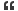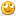Thread: convert one dimansional array to two dimansional array

1. convert one dimansional array to two dimansional array

Question asked by visitor Ms.Supriya Ahire.

Hello All,

Plz send me solution for following question...
program to convert one dimansional array to two dimansional array .

Thanks & Regards,
Ms.Supriya Ahire.Reply With Quote

2. Re: convert one dimansional array to two dimansional array

two dimenation mean second dimention indicates some field which will vary depends on user ?

do you guess what the user give the size of second dimention?Reply With Quote

3. Re: convert one dimansional array to two dimansional array

suppose we do the following c code

int a,i;

here i declared a is an one dimentional array . After compilation it is not possible to convert into two dimensional .Reply With Quote

4. Re: convert one dimansional array to two dimansional array

if the single dimentional array has to 5 elements like
int a, which stores elements in single row.
but in two dimentional we have row and coloumn. now we change above in to two dimentional
int a[];
the declaration tells a is a variable of type integer which has 5 rows and 0 coloumns and now write your program in two dimentional.Reply With Quote

5.Re: convert one dimansional array to two dimansional arrayOriginally Posted by rahulvegiif the single dimentional array has to 5 elements like
int a, which stores elements in single row.
but in two dimentional we have row and coloumn. now we change above in to two dimentional
int a[];
the declaration tells a is a variable of type integer which has 5 rows and 0 coloumns and now write your program in two dimentional.
thnks...Reply With Quote

6. Re: convert one dimansional array to two dimansional arrayOriginally Posted by Geek_GuestQuestion asked by visitor Ms.Supriya Ahire.

Hello All,

Plz send me solution for following question...
program to convert one dimansional array to two dimansional array .

Thanks & Regards,
Ms.Supriya Ahire.
Supriya,

You need to clarify this question better. Lets consider an example.
1.
int arr_of_colors = {red, orange, black};
This is a One-dimensional array.

2.
int favorite_color [person][favorite color];
This is a two dimensional array: one dimension is the person, and second dimension is the favorite color.

3.
So, would it make sense to say "convert one dimensional array to a two dimensional array??" It does not to me. So, can you clarify your question??Reply With Quote

7. convert one dimansional array to two dimansional array

Part i: statically allocated two dimensional arrays are defined using a syntax of type arrayname [rows][cols]; they are accessed in the same way as one-dimensional arrays: arrayname[r][c] accesses the value at row r, column c. Write a program that creates a two-dimensional array of integers with 5 rows and 3 columns, initializes each value to 0, and then writes out the array, row by row, one row per line. Passing multidimensional arrays is a little bit harder than passing one-dimensional arrays. Here is a sample header: const int numcolumns=15; // possibly more code here... Void foo(int my2darray[][numcolumns]) // notice we must tell how // many columns in the array // but must not how many rows... { // the code } main() { int myarray[numcolumns]; foo(myarray); } modify your previous program to pass its array to an initialize function that initializes the array, and to a print function that outputs the array. Handin problem 1: as with one-dimensional arrays, it is annoying to require different syntax for declaring and passing the arrays. Modify your array.h and array.c solutions (from cs 180) to work for a two-dimensional array (e.g. Create an array2d class). You will need constants for maxrows, maxcolumns, and class variables for numrows, numcoluns (as opposed to just length). Test your program by modifying the previous program to work with your new class. Now u happy . Why u waste time in sending mails , go to google search man .... Bye.. Have a nice dayReply With Quote

8.Posting Permissions

• You may not post new threads
• You may not post replies
• You may not post attachments
• You may not edit your posts
•